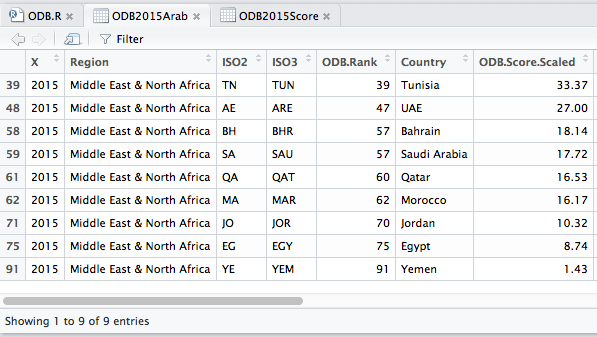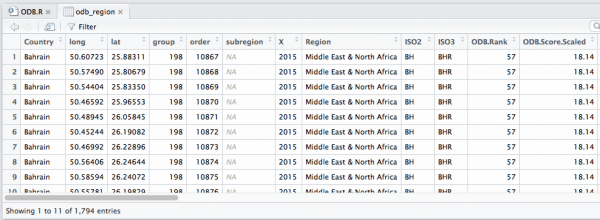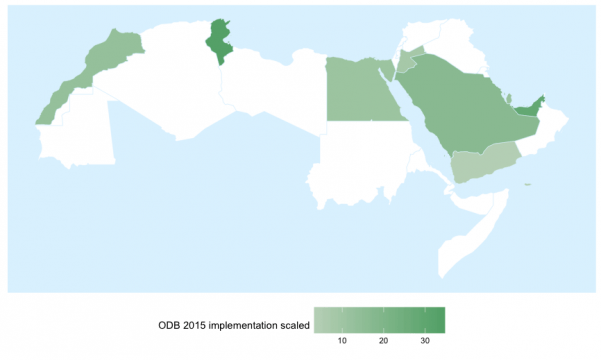May 20, 2016

# How to Create Open Data regional Heatmap with RIn the regional Open Data report in Arab world, the map in the report cover was created in R. Looks simple ? Let’s see how to do this :

First we need some data, so we have to download the ODB-3rdEdition-Rankings.csv

```ODB2015Score <- read.csv('http://opendatabarometer.org/data/3rdEdition/ODB-3rdEdition-Rankings.csv')
View(ODB2015Score)
```I’ll use here the IDs to select the 9 Arab countries using :

```ODB2015Arab <- ODB2015Score[c(39,48,58,59,61,62,71,75,91),]
View(ODB2015Arab)```That’s pretty simple I think ! We got the data, now let’s put this on a map. I’ll be using ggplot2 library here, so we’ll need to install.package first if you don’t have it already :

```install.packages('ggplot2') ## install the library
library('ggplot2') ## Load the library ggplot2
world <- map_data('world') ## World map data
ggplot(world,aes(x=long,y=lat,group=group))+geom_path() ## Plot the world map```We don’t need to plot the whole world, the reason why we have to use a subset, I’ll put the whole mena_region in one variable, then use odb_region to put only countries that are covered in this edition of the ODB.

```arab_region <- c('Somalia','Eritrea','Western Sahara','Mauritania','Sudan','Algeria', 'Bahrain', 'Djibouti', 'Egypt', 'Iraq', 'Jordan', 'Kuwait', 'Lebanon', 'Libya', 'Malta', 'Morocco', 'Oman', 'Qatar', 'Saudi Arabia', 'Syria', 'Tunisia', 'United Arab Emirates', 'Palestine', 'Yemen')
mena_region = subset(world,region %in% arab_region)
odb_region = subset(world, region %in% ODB2015Arab\$Country)
ggplot(odb_region,aes(x=long,y=lat,group=group))+geom_path()```Here you should notice that there is one missing country in the map (That I did not notice myself in the beginning too), can you notice it ? Well in the ODB document Emirates is mentioned as UAE while in the map_data it’s mentioned as United Arab Emirates, so we’ll need to fix this :This is the behaviour of read.csv which convert string to factor, simple way to fix this is to disable stringsAsFactors since the beginning, the whole code become :

```ODB2015Score <- read.csv('http://opendatabarometer.org/data/3rdEdition/ODB-3rdEdition-Rankings.csv',stringsAsFactors = FALSE)
ODB2015Arab <- ODB2015Score[c(39,48,58,59,61,62,71,75,91),]
ODB2015Arab[2,6] = 'United Arab Emirates'
library('ggplot2')
world <- map_data('world')
arab_region <- c('Somalia','Eritrea','Western Sahara','Mauritania','Sudan','Algeria', 'Bahrain', 'Djibouti', 'Egypt', 'Iraq', 'Jordan', 'Kuwait', 'Lebanon', 'Libya', 'Malta', 'Morocco', 'Oman', 'Qatar', 'Saudi Arabia', 'Syria', 'Tunisia', 'United Arab Emirates', 'Palestine', 'Yemen')
arab_region = subset(world,region %in% arab_region)
odb_region = subset(world, region %in% ODB2015Arab\$Country)
ggplot(odb_region,aes(x=long,y=lat,group=group))+geom_path()
```I can see the missing country ! Well, I that’s not the exact code but you should notice that all countries are there !

Now I will plot the ODB data on this map, first thing is to merge odb_region with ODB2015Arab :

```names(odb_region) <- c('long','lat','group','order','Country','subregion')
odb_region <- merge(odb_region,ODB2015Arab,by = "Country")```

I just renamed the ‘region’ column to be able to merge it by ‘Country’. See the result below :You notice that odb_region have 31 variables after it was only 6 before the merge. Now you can plot any variable available in ODB-3rdEdition-Rankings.csv file.

Let’s start with the ODB Score, the one I used on the report cover, I think the code below is self-explanatory :

```p<-ggplot()
p <- p+geom_polygon(data=odb_mena, aes(x=long,y=lat,group=group),fill='white',color='#DFF3FE',size=0.2) ## Plot and fill the mena_region in white

p<- p+  geom_polygon(data = odb_region, aes(x=long,y=lat,group=group, fill=ODB.Score.Scaled), size = 0.2, color='#DFF3FE') + # Plot odb_region, fill based on ODB Score variable
scale_fill_gradient(low="#A7CD7B", high="#567E2B", name='ODB 2015 score scaled') + # Gradient params, you can play with the low and high colours here
theme(
legend.position = 'none',
legend.key.size = unit(1, "cm")
) +
theme(panel.background = element_rect(fill = "#DFF3FE"), panel.grid = element_blank())
p<- p+ theme(axis.title.x=element_blank(),
axis.text.x=element_blank(),
axis.ticks.x=element_blank())
p<- p+theme(axis.title.y=element_blank(),
axis.text.y=element_blank(),
axis.ticks.y=element_blank())
p```I will not go into explaining the whole code in details as it’s just parameters of the ggplot() function to remove axis, labels, set colors.. etc. We can plot for example the Rank.Change this way  :Or we the Implementation :The only issue here is how to make the right choice of colours. Hope this could help you to play with ODB data and plot any kind of variable in the region of your choice.

Enjoy !